# Electron Energy Distribution Function

August 4, 2014

The electron energy distribution function (EEDF) plays an important role in plasma modeling. Various approaches can be used to describe the EEDF, such as Maxwellian, Druyvesteyn, or using a solution of the Boltzmann equation. Today, we will demonstrate the influence the EEDF has on a plasma model’s results. Additionally, we present a way to compute the EEDF with the Boltzmann Equation, Two-Term Approximation interface.

### Electron Energy Distribution Function (EEDF)

The electron energy distribution function (EEDF) is essential in plasma modeling because it is needed to compute reaction rates for electron collision reactions. Because electron transport properties can also be derived from the EEDF, the choice of the EEDF you use influences the results of the plasma model. If the plasma is in thermodynamic equilibrium, the EEDF has a Maxwellian shape. In most plasmas, for technical purposes, deviations from the Maxwellian form occur.

### Describing the EEDF

To describe the EEDF, several possibilities are available, such as a Maxwell or Druyvesteyn function. In addition, a generalized form is available, which is an intermediate between the Maxwell and the Druyvesteyn function.

#### Functions That Describe the EEDF

 Maxwell f(\epsilon)=\varphi^{-3/2}\beta_1\exp\left(-\frac{\epsilon\beta_2}{\varphi}\right) \beta_1=\Gamma(5/2)^{3/2}\Gamma(3/2)^{-5/2},\ \beta_2=\Gamma(5/2)\Gamma(3/2)^{-1} Druyvesteyn f(\epsilon)=\varphi^{-3/2}\beta_1\exp\left(-\left(\frac{\epsilon\beta_2}{\varphi}\right)^2\right) \beta_1=\Gamma(5/4)^{3/2}\Gamma(3/4)^{-5/2},\ \beta_2=\Gamma(5/4)\Gamma(3/4)^{-1} Generalized f(\epsilon)=\varphi^{-3/2}\beta_1\exp\left(-\left(\frac{\epsilon\beta_2}{\varphi}\right)^g\right) \beta_1=\Gamma(5/2g)^{3/2}\Gamma(3/2g)^{-5/2},\ \beta_2=\Gamma(5/2g)\Gamma(3/2g)^{-1}

Here, ϵ is the electron energy, (eV); \varphi is the mean electron energy, (eV); and g is a factor between 1 and 2. For a Maxwell distribution function, g is equal to 1, while g equals 2 for a Druyvesteyn distribution. Lastly, \Gamma is the incomplete Gamma function.

#### Druyvesteyn

As the Druyvesteyn EEDF is based on a constant (electron energy independent) cross section, the Maxwellian EEDF is based on constant collision frequency. The distribution functions assume that elastic collisions dominate, thus the effect of inelastic collisions (e.g., excitation or ionization) on the distribution function is insignificant. In such a case, the distribution function becomes spherically symmetric. In elastic collisions with neutral atoms, the electrons’ direction of motion is changed, but not their energies (due to the large mass difference).

#### Maxwellian

If the electrons are in thermodynamic equilibrium among each other, the distribution function is Maxwellian. However, this is only true if the ionization degree is high. Here, electron-electron collisions drive the distribution towards a Maxwellian shape. Inelastic collisions of electrons with heavy particles lead to a drop of the EEDF at higher electron energies. Therefore, a Druyvesteyn distribution function often gives more accurate results for a lower ionization degree.

#### The Boltzmann Equation

Furthermore, the EEDF can be computed by solving the Boltzmann equation. The Boltzmann equation describes the evolution of the distribution function, f, in a six-dimensional phase space:

\frac{\partial f}{\partial t}+\mathbf{v}\cdot\triangledown f-\frac{e}{m}(\mathbf{E}\cdot\triangledown_\mathbf{v}f)=C[f]

To solve the Boltzmann equation and, therefore, compute the EEDF, drastic simplifications are necessary. A common approach is to expand the distribution function in spherical harmonics. The EEDF is assumed to be almost spherically symmetric, so the series can be truncated after the second term (a so-called two-term approximation). This approach is the most accurate way to compute the EEDF because an anisotropic perturbation, due to inelastic collisions, is taken into account. However, this is also the most computationally expensive approach.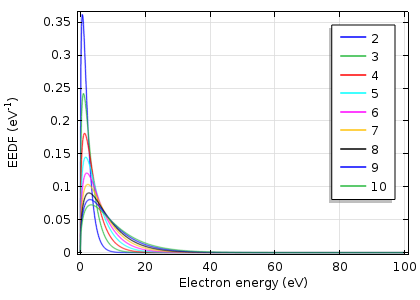Maxwellian EEDF in eV–1 for mean electron energies from 2 — 10 eV.

Normally, the distribution function is divided by \sqrt{\epsilon} for illustration purposes. This kind of distribution function is also known as an electron energy probability function (EEPF). For a Maxwellian function, this results in a straight line with a slope of (-1/k_B T), as shown below.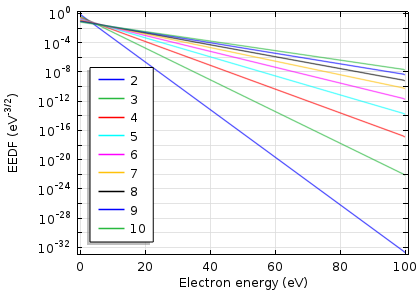Maxwellian EEPF in eV–3/2 for mean electron energies from 2 — 10 eV.

### Comparing the Maxwell, Druyvesteyn, and Boltzmann Distribution Functions

A Druyvesteyn distribution has the maximum and mean energy shifted to higher values. The high-energy tail drops much faster in comparison to a Maxwellian distribution. As the electrons reach enough energy for excitation or ionization, inelastic collisions occur. This leads to the fall of the Boltzmann distribution function, which is observed below.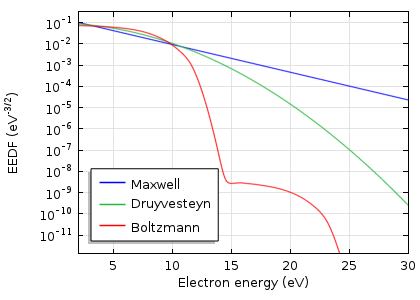Comparison of a Maxwell, Druyvesteyn, and Boltzmann distribution function.
Mean electron energy 5 eV, electron density 1\cdot10^{16}\ \mathrm{m}^{-3}, ionization degree 1\cdot10^{-9}

### Influencing the Model Results

In the plasma model, the EEDF is needed to compute the rate coefficients, k_k, for the electron collisions reactions, according to the equation:

k_k=\gamma\int\limits_{0}^{\infty}\epsilon\sigma_k(\epsilon)f(\epsilon)\mathrm d\epsilon

In the above equation, \gamma = \sqrt{2q/m_e}, the electron energy is ϵ, and \sigma_k is the cross section for reaction, k.

The rate coefficients for excitation and ionization highly depend on the shape of the EEDF. This is due to the exponential drop-off in the population of electrons at energies exceeding the activation threshold. Using a Maxwellian EEDF can lead to an overestimation of the ionization rate, which is shown below.Rate coefficients for argon ionization computed with different kinds of EEDFs.

Furthermore, the electron transport properties can be computed by means of the EEDF, using the Boltzmann Equation, Two-Term Approximation interface. The computed transport coefficients have less dependence on the type of EEDF.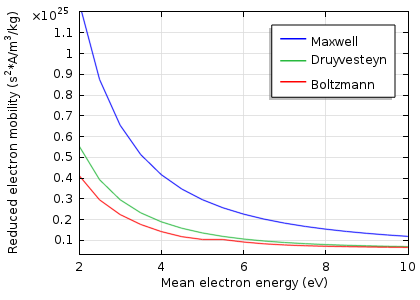Reduced electron mobility computed with different kinds of EEDFs.

### Comparing the Model Results of a Dielectric Barrier Discharge

As the rate coefficients can differ by orders of magnitude, we must be aware that the discharge characteristics also change drastically when changing the EEDF. In the Plasma Module Model Library, there is a model of dielectric barrier discharge (DBD). This model simulates electrical breakdown in atmospheric pressure argon. The model was recomputed with the three different kinds of EEDFs, and we compared the results. The next two figures show the total current at the grounded electrode and the instantaneously absorbed power in the plasma. The plasma is driven with a sinusoidal voltage with a frequency of 50 kHz. The figures show the behavior over two periods.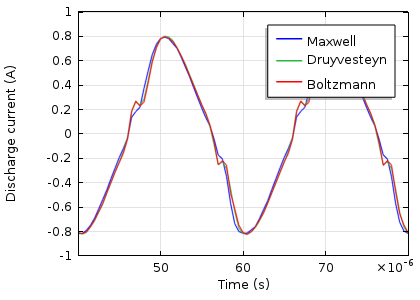Total discharge current in the DBD vs. time.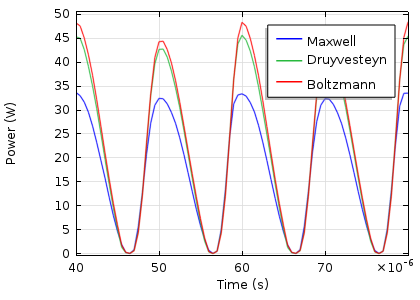Absorbed power of the DBD vs. time.

The results look quite similar. So, the choice of the EEDF influences the modeling results, not orders of magnitude, but in this case much less than a factor of two. This, of course, depends on the model and the specific results you wish to extract.